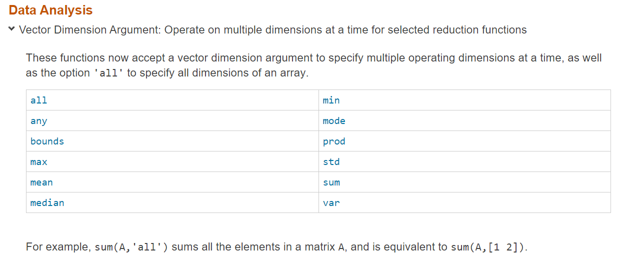# dimsum in MATLAB10

Posted by Loren Shure,

As Steve noted in a recent post, several of the functions that operate along specific dimensions have been updated to add some new functionality, for example the function sum. (I also think he missed a great opportunity for a cool blog post title, which is only partly why I am writing about it now :^) ). Please read his post!### Contents

#### Summing Elements of an Array

As you likely know, MATLAB has a collection of functions that operate on inputs, treating the effective column vectors as individual units. There functions include sum, prod, cumsum, mean, to name a few.

Originally, you could only use these functions with a single input. And the operation (+, *, etc.) would occur along the first non-singleton dimension. This tripped people up enough and was inconvenient enough that we eventually added an optional dimension argument. And, if you wanted to apply the operation to the entire array, we taught you to string the input into a long column vector (A(:)) and apply the necessary function to this transformed array.

#### An Aside - the Engineering Rule of Thumb

We had a wonderful engineer here many years ago that taught me the "engineering rule of thumb". Either you disallow something always, you allow it once, or you always allow it. This got shortened to the "0, 1, or infinity rule". Look at the case of sum as a representative function. If you simply require the default behavior, you need not supply any additional arguments beyond the array itself (0), you can use an optional scalar argument (1) to specify the dimension to work on, and now you can have that optional argument be a vector of dimensions (infinity, theoretically - but you'll never fit that into MATLAB!).

#### Back to Dimensions

In R2018b we added a perhaps friendlier syntax for the func(A(:)) syntax, now func(A,'all'). This is in addition to allowing no dimension input, a scalar dimension input, or a vector of dimensions - 0, 1, infinity.

Here's the current list of functions to which these new input patterns apply.Is this new feature helpful to you? Let us know here.

Get the MATLAB code

Published with MATLAB® R2019a

### Note

Roman Voronov replied on : 1 of 10
Yes, it is helpful. I think I asked for it via a feature request like 10 yrs ago. Lol
Andrew Stamps replied on : 2 of 10
Which, of course, now allows you to write:
y = all(A, 'all');
Only mildly confusing. ;-)
Loren Shure replied on : 3 of 10
@Andrew - I had said this (but that's because you decided to create a variable named all. Not a great idea.) in sleep deprived state. Sorry! Yes, that code does look odd! @Roman - never say never :-) !
Kevin van de Ruit replied on : 4 of 10
How about allowing vectors as second input in size?
Loren Shure replied on : 5 of 10
@Kevin- That's also part of the new syntax - you can see it if you look at the help. Here's the synopsis:
S = sum(A)
S = sum(A,'all')
S = sum(A,dim)
S = sum(A,vecdim)
S = sum(___,outtype)
S = sum(___,nanflag)

madhan ravi replied on : 6 of 10
A really interesting blog indeed. Hoping to see a function just like pagefun() which just performs 3D matrix multiply between matrices. At the moment, it's possible by looping through the third dimension and performing the operation, is there any other alternative/s ?
Loren Shure replied on : 7 of 10
@Madhan- I believe there's something on our wishlist. Best if place an enhancement request for what you want, as specific an example as possible of what you are trying to achieve. Thanks! --loren
madhan ravi replied on : 8 of 10
First of all thank you very much for your reply Loren.I am a huge fan of your blogs. To illustrate what I mean , here is an example:
A = zeros(2,2,2); % consider two 3D matrices
A(:,:,1) = [1:2;3:4];
A(:,:,2) = 2*[1:2;3:4];
B = zeros(2,2,2);
B(:,:,1) = 2*[1:2;3:4];
B(:,:,2) = 3*[1:2;3:4];
Wanted = zeros(size(A));
% 3D matrix multiply
for k = 1:size(A,3)
Wanted(:,:,k) = A(:,:,k) * B(:,:,k); % performing matrix multiplication
end
% Which gives
Wanted(:,:,1) =

14    20
30    44

Wanted(:,:,2) =

42    60
90   132
%So to perform the above operation let's consider the existing function mtimes() it would be a lot cooler just to simply use
mtimes(A,B,3)
%          ^---- just denoting the third dimension

I hope , I am clear now.                
Loren Shure replied on : 9 of 10
Thanks, Madhan, Yes, you were clear before. For development to push for a feature, it helps if users ask for it via a tech support enhancement request that gets logged with that sort of info. If you could enter that into the system, it would be awesome. Thanks! --loren
madhan ravi replied on : 10 of 10
Thank you , Loren. Will sure do.